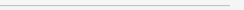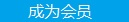要资料 文章 文库 视频 Code iProcess 课程 角色 咨询 工具 火云堂 讲座吧 成长之路每天15篇文章不仅获得谋生技能 更可以追随信仰分享到

 `root# g++ test_bgl.cpp I/usr/boost/boost_1_44/ -L/usr/boost/boost_1_44/lib `

BGL 可从 Boost 网站免费下载（请参阅 参考资料，获取有关的链接）。BGL 是一个仅有头文件的库，因此，以后在应用程序代码中使用该库时，需要在源代码中包含相关的头文件。但是 BGL 需要这个序列化库来进行链接。以下是一个典型的命令行格式：

 `template class adjacency_list { }; `

OutEdgeList 模板参数决定了将用于存储边列表（ edge-list）信息的容器类型。回顾一下图论基础知识就可以知道，对于有向图，只具有入边的那些顶点都有一个对应的空邻接表。默认值被设置为 vecS，该值对应于 std::vector。VertexList 模板参数决定了用于表示该图顶点列表的容器类型，默认值同样被设置为 std::vector。DirectedS 模板参数根据提供的值是 directedS 还是 undirectedS 来确定该图是有向图还是无向图。

 `#include using namespace boost; typedef boost::adjacency_list mygraph; int main() { mygraph g; add_edge (0, 1, g); add_edge (0, 3, g); add_edge (1, 2, g); add_edge (2, 3, g); }`

 `#include using namespace boost; typedef boost::adjacency_list mygraph; int main() { mygraph g; add_edge (0, 1, g); add_edge (0, 3, g); add_edge (1, 2, g); add_edge (2, 3, g); mygraph::vertex_iterator vertexIt, vertexEnd; mygraph::adjacency_iterator neighbourIt, neighbourEnd; tie(vertexIt, vertexEnd) = vertices(g); for (; vertexIt != vertexEnd; ++vertexIt) { cout << *vertexIt << " is connected with "; tie(neighbourIt, neighbourEnd) = adjacent_vertices(*vertexIt, g); for (; neighbourIt != neighbourEnd; ++neighbourIt) cout << *neighbourIt << " "; cout << "\n"; }} `

BGL 的优势之一是它是高度可配置的。BGL 允许您使用下列任何选择器类型来配置顶点集和边集合，这些选择器类型都是在头文件中定义的；您需要做的就是在声明图时使用它们：

• vecS 选择 std::vector
• lists 适用于 std::list
• slistS 选择 std::slist
• setS 选择 std::set
• multiSetS 选择 std::multiset
• hash_setS 选择 std::hash_set

 `#include using namespace boost; typedef boost::adjacency_list mygraph; int main() { mygraph g; add_edge (0, 1, g); add_edge (0, 3, g); add_edge (1, 2, g); add_edge (2, 3, g); // Same as Listing 3`

 `#include #include using namespace boost; using namespace std;int main( ){ undirected_graph<>g; undirected_graph<>:vertex_descriptor u = g.add_vertex(); undirected_graph<>:vertex_descriptor v = g.add_vertex(); undirected_graph<>:vertex_descriptor w = g.add_vertex(); undirected_graph<>:vertex_descriptor x = g.add_vertex(); add_edge(u, v, g); add_edge(u, w, g); add_edge(u, x, g); cout << "Degree of u: " << degree(u, g); return 0; } `

 `template < typename VertexProp = no_property, typename EdgeProp = no_property, typename GraphProp = no_property> class undirected_graph { // public: typedef adjacency_list graph_type; private: graph_type m_graph; // }; `

 `#include using namespace boost; typedef boost::adjacency_list mygraph; int main() { mygraph g; add_edge (0, 1, g); add_edge (0, 3, g); add_edge (1, 2, g); add_edge (2, 3, g); mygraph::vertex_iterator vertexIt, vertexEnd; mygraph::in_edge_iterator inedgeIt, inedgeEnd; mygraph::in_edge_iterator outedgeIt, outedgeEnd; tie(vertexIt, vertexEnd) = vertices(g); for (; vertexIt != vertexEnd; ++vertexIt) { cout << "incoming edges for " << *vertexIt << ": "; tie(inedgeIt, inedgeEnd) = in_edges(*vertexIt, g); for(; inedgeIt != inedgeEnd; ++inedgeIt) { cout << *inedgeIt << " "; } cout << "\n"; } for (; vertexIt != vertexEnd; ++vertexIt) { std::cout << "out-edges for " << *vertexIt << : ; tie(outedgeIt, outedgeEnd) = out_edges(*vertexIt, g); // Similar to incoming edges } } `

• std::pair<edge_iterator, edge_iterator> edges(const adjacency_list& g)：返回图 g 中边的相对应迭代程序
• vertices_size_type num_vertices(const adjacency_list& g)： 返回图 g 中顶点的数量
• edges_size_type num_edges(const adjacency_list& g)：返回图 g 中边的数量
• vertex_descriptor source(edge_descriptor e, const adjacency_list& g)：返回一条边的源顶点
• vertex_descriptor target(edge_descriptor e, const adjacency_list& g)：返回一条边的目标顶点
• degree_size_type in_degree(vertex_descriptor u, const adjacency_list& g)：返回一个顶点的入度 (in-degree)
• degree_size_type out_degree(vertex_descriptor u, const adjacency_list& g)：返回一个顶点的出度 (out-degree)

 `// usual typedefs here, refer to previous listings int main() { mygraph g; add_edge (0, 1, 8, g); add_edge (0, 3, 18, g); add_edge (1, 2, 20, g); add_edge (2, 3, 2, g); add_edge (3, 1, 1, g); add_edge (1, 3, 7, g); cout << "Number of edges: " << num_edges(g) << "\n"; cout << "Number of vertices: " << num_vertices(g) << "\n"; mygraph::vertex_iterator vertexIt, vertexEnd; tie(vertexIt, vertexEnd) = vertices(g); for (; vertexIt != vertexEnd; ++vertexIt) { std::cout << "in-degree for " << *vertexIt << ": " << in_degree(*vertexIt, g) << "\n"; std::cout << "out-degree for " << *vertexIt << ": " << out_degree(*vertexIt, g) << "\n"; } mygraph::edge_iterator edgeIt, edgeEnd; tie(edgeIt, edgeEnd) = edges(g); for (; edgeIt!= edgeEnd; ++edgeIt) { std::cout << "edge " << source(*edgeIt, g) << "-->" << target(*edgeIt, g) << "\n"; } } `

• void remove_edge(vertex_descriptor u, vertex_descriptor v, adjacency_list& g)：从图 g 中删除一条边
• void remove_edge(edge_descriptor e, adjacency_list& g)：从图 g 中删除一条边
• void clear_vertex(vertex_descriptor u, adjacency_list& g)： 删除顶点 u 的所有边
• void clear_out_edges(vertex_descriptor u, adjacency_list& g)：删除有向图 g 中顶点 u 的所有出边（不适用于无向图）
• void clear_in_edges(vertex_descriptor u, adjacency_list& g)： 删除有向图 g 中顶点 u 的所有入边（不适用于无向图）
• void remove_vertex(vertex_descriptor u, adjacency_list& g)： 从图 g 中删除一个顶点（如果已使用 clear_vertex 或其他适当函数删除与该定顶点相关的所有边。）

property 是一个可分配给顶点和边的参数。您可以使用一个标签名和一个与 property 相关的类型来定义该属性。BGL 有几个可用的标签名，其中包括 edge_weight_t 和 vertex_name_t。例如，要在图的顶点中存储标签名，可以将一个 property 定义为 typedef property<vertex_name_t, std::string> VertexNameProperty，然后将该属性传递给图的模板声明中的 VertexProperty 参数。

 `typedef property EdgeWeightProperty; `

 `typedef boost::adjacency_list mygraph; `

 `#include using namespace boost; typedef property EdgeWeightProperty; typedef boost::adjacency_list mygraph; int main() { mygraph g; add_edge (0, 1, 8, g); add_edge (0, 3, 18, g); add_edge (1, 2, 20, g); add_edge (2, 3, 2, g); add_edge (3, 1, 1, g); add_edge (1, 3, 7, g); } `

BGL 中的最小生成树（spanning tree

BGL 最精彩地方的就是有大量可用于图的预定义算法：Kruskal、Prim、Dijkstra 等，凡是您说得出的，BGL 都有。修改 清单 8 中的代码，从而拥有一个具有加权边的无向图，然后使用 Kruskal 算法得到最小生成树，此时您就会明白我的意思了。BGL 将每个算法放在不同的头文件中，因此，要使用 Kruskal 算法，必须包含 boost/graph/kruskal_min_spanning_tree.hpp 头文件。清单 9 展示了相关代码。

 `#include // typedef boost::adjacency_list mygraph; typedef mygraph::edge_descriptor Edge; int main() { mygraph g; add_edge (0, 1, 8, g); add_edge (0, 3, 18, g); add_edge (1, 2, 20, g); add_edge (2, 3, 2, g); add_edge (3, 1, 1, g); add_edge (1, 3, 7, g); std::list < Edge > spanning_tree; kruskal_minimum_spanning_tree (g, std::back_inserter(spanning_tree)); for (std::list < Edge >::iterator ei = spanning_tree.begin(); ei != spanning_tree.end(); ++ei) { cout << *ei << " "; } cout << "\n"; } `

BGL 中的访客（visitor） 在 STL 中充当仿函数角色，除此之外，它还可以做很多事情。访客没有 operator() 之类的单个方法，但可以灵活地定义几种方法，比如 initialize_index、start_index、discover_index 和 examine_edge。毫不夸张地说，BGL 通过提供这些 hook 函数可以让您定制 DFS。首先我们看一个使用 DFS 的样例代码（参见 清单 10）。

 ` #include #include #include using namespace std; using namespace boost; typedef property EdgeWeightProperty; typedef boost::adjacency_list < listS, vecS, undirectedS, no_property, EdgeWeightProperty> mygraph; class custom_dfs_visitor : public boost::default_dfs_visitor { public: template < typename Vertex, typename Graph > void discover_vertex(Vertex u, const Graph & g) const { std::cout << "At " << u << std::endl; } template < typename Edge, typename Graph > void examine_edge(Edge e, const Graph& g) const { std::cout << "Examining edges " << e << std::endl; } }; int main() { mygraph g; add_edge (0, 1, 8, g); add_edge (0, 3, 18, g); add_edge (1, 2, 20, g); add_edge (2, 3, 2, g); add_edge (3, 1, 1, g); add_edge (1, 3, 7, g); custom_dfs_visitor vis; depth_first_search(g, visitor(vis));} `

Hook 函数 用途
start_vertex(u, g) 在开始遍历之前调用源顶点
discover_vertex(u, g) 第一次调用顶点时调用
finish_vertex(u, g) 如果 u 是一个树的根节点，则在调用该树上其他所有元素之后调用 finish_vertex。如果 u 是一个叶子节点，则在完成 u 所有出边的检查之后调用该方法。
examine_edge(u, g) 找到顶点 u 后，调用其每个出边
tree_edge(u, g) 当一条边成为搜索树的边时调用
back_edge(u, g) 调用一个图的回边（back edges）；用于无向图，因为 (u, v) 和 (v, u) 是同一条边，所以 tree_edge 和 back_edge 均被调用

 Visual C++编程命名规则 任何时候都适用的20个C++技巧 C语言进阶 串口驱动分析 轻轻松松从C一路走到C++ C++编程思想

 C++并发处理+单元测试 C++程序开发 C++高级编程 C/C++开发 C++设计模式 C/C++单元测试

 北京 嵌入式C高质量编程 中国航空 嵌入式C高质量编程 华为 C++高级编程 北京 C++高级编程 丹佛斯 C++高级编程 北大方正 C语言单元测试 罗克韦尔 C++单元测试每天2个文档/视频 扫描微信二维码订阅
 订阅技术月刊 获得每月300个技术资源